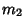## Geometrography

A quantitative measure of the simplicity of a Geometric Construction which reduces geometric constructions to five steps. It was devised by È. Lemoine.Place a Straightedge's Edge through a given Point,Draw a straight Line,Place a Point of a Compass on a given Point,Place a Point of a Compass on an indeterminate Point on a Line,Draw a Circle.

Geometrography seeks to reduce the number of operations (called the ``Simplicity'') needed to effect a construction. If the number of the above operations are denoted,,,, and, respectively, then the Simplicity isand the symbol is. It is apparently an unsolved problem to determine if a given Geometric Construction is of the smallest possible simplicity.